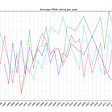# Quickly Master the Principal Components Analysis: the Data Dimensionality Reduction Technique You Must Know

Why does dimensionality reduction matter?

The higher the data dimension, the more difficult it is to be fitted by a model. Let’s take the modeling of linear regression as an example. When it includes two predictors and one target, it is easy to conclude that the “space” for the model-fitting process is loosely x times y, which is a quadratic variable.

Then one more predictor is added. Now, the “space” for the model-fitting process turns to be loosely x times y times z, which is a cubic variable.

To summarize, although the dimension of data here only increased 50% (i.e., from 2 to 3), the capacity of the “space” ran through exponential growth (i.e, from quadratic to cubic), which means the difficulty of the model-fitting process increases dramatically.

How does the principal components analysis (PCA) work for dimension reduction?

The PCA reallocates the total variance of predictors into the “components”, leading the distribution of the variance to be more concentrated. Let’s consider the scenario of the linear regression. When it includes two predictors and one target, applying the PCA would provide us with the same number of “components” as well. What makes the component differ from the original predictor is that the less number of components will include more variance than that of original predictors. In other words, given two predictors for model-fitting, it is possible to reach the same model performance by using only one component.

# Dive into the PCA by a Super Easy Example

Suppose that there are three predictors as shown below ready for modeling:

The variance of each predictor has been provided, and the sum of variance is 21.1088. Then we applied the PCA by the following python code, and we acquired three components as expected.

`# Applying the PCA to the example import numpy as npfrom sklearn.decomposition import PCApredictors = np.array([[10.5, 11.2, 8.9],[7.3, 5.6, 3.2], [4.2, 8.1, 9.0],[10.4, 3.2, 7.6]])# create a 'pca' instance with 3 componentspca = PCA(n_components=3)pca.fit(predictors)# show all 3 componentspca.transform(predictors)`

As we could see here, both the total variance of components and predictors are exactly the same! Surprisingly, the first and the second component have 14% more variance than that of X1 and X2.

Thus, we could find that though the total amount of variance is constant, it has been concentrated into these top components. Now, if we decided to apply the first two components from the PCA for modeling, we should not suffer from losing a lot of information from three original predictors.

--

--

--

## More from MLearning.ai

Data Scientists must think like an artist when finding a solution when creating a piece of code. ⚪️ Artists enjoy working on interesting problems, even if there is no obvious answer ⚪️ linktr.ee/mlearning 🔵 Follow to join our 18K+ Unique DAILY Readers 🟠

## Running CapsuleNet on TensorFlow## Suicidal Tendencies Detection With Machine Learning: Data Preprocessing## Five Assumptions Of Linear Models## Exploring Undernourishment: Part 8 — Recommendations and Conclusions## Anomaly Detection Part 2: The Bigeye Approach## Day 9 of #66DaysOfDataChallenge## 15 Stunning Data Visualizations (And What You Can Learn From Them)## Plotting IMDb Average Rating## Haozhou Zhou

Data Science Enthusiast | To be a bonafide Guitarist

## How to Make Systematic Choices of Machine Learning Models## Feature Transformation## Decision Trees — First step towards Classification!## Supervised learning Vs unsupervised learning